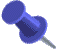## Gordon Nipp's Tables of Quinary Quadratic FormsKeywords: tables, reduced, regular, primitive, positive definite, quinary, quadratic forms, five-dimensional lattices, automorphism group, mass, genus, genera, Hasse symbolThese tables were computed by Gordon L. Nipp (gnipp@calstatela.edu), of the Department of Mathematics, California State University, Los Angeles, CA 90032, USA, and are included here with his permission.## Contents of these files## Format of these files

These are tables of reduced regular primitive positive-definite quinary quadratic forms over the rational integers.

They were computed by Gordon L. Nipp (see above) and are included here with his permission.

These tables contain one 5-variable quadratic form from each primitive class of such forms through discriminant d=513. The classes are grouped into genera; the mass of each genus has been computed, and, for each prime p dividing 2d, Hasse symbols are given. In addition, the number of automorphs for each form has been computed, so that the well-known relationship between the mass of the genus and the number of automorphs of each class serves as a check on the accuracy of the tables.

Based on results of Minkowski  and van der Waerden  and beginning with the quaternary forms through discriminant 1296 contained in Nipp  (ample to ensure completeness), we generated successive large collections of reduced quinary forms containing forms in all possible quinary classes through d=513 (the discriminant is that of Watson ). Grouping into classes and choosing a representative of each class was accomplished using a well-tested computer program described for the quaternary case in , and a similar program was used to compute the number of automorphs for each such representative. The classes were then separated into genera using an upgrade of a program described in  and based on results contained in O'Meara's book . The 2-adic density a_2 of each form was computed as in Watson's article , and for primes p dividing d, p >2, p-adic densities a-p were calculated using Pall's results . Following Siegel  and Pall , the mass was computed according to the formula

d^3 Product (1-p^{-2})(1-p^{-4}), p|d, p>2
M =     --------------------------------------------------
128 Product a_p, p|2d

As an example of the format of the tables, we include the eleventh (of twelve) genera of discriminant 120:

``` D= 120; GENUS#   11; MASS=   5/   64; HASSE SYMBOLS ARE   1-1-1
1   1   1   3   4   0   0   0   0   0   0   1   1   1   3;   192
1   2   2   2   3   0   0   0   1   2   2   0   0   2   1;    32
1   2   2   2   3   0   0   2   1   2   0   0   2   0   1;    24
```
Here the mass of the genus is 5/64, the Hasse symbols are 1, -1, and -1 at primes 2, 3, and 5 respectively, and the three classes in the genus have 192, 32, and 24 automorphs respectively. The first class is represented by the form

x11^2 + x22^2 + x33^2 + 3x44^2 + 4x55^2 + x1x5 + x2x5 + x3x5 + 3x4x5

In general, the coefficients for a quinary form are given in the order a11,a22,a33,a44,a55,a12,a13,a23,a14,a24,a34,a15,a25,a35,a45 where aij is the coefficient of xixj. As a check one notes that the mass, computed using the formula above, is 5/64=1/192+1/32+1/24, the sum over the classes of the reciprocals of the number of automorphs.

The computations were performed on the California State University Cyber 960 and on the CSU Sacramento Multiflow Trace machine.

### References

 H. Minkowski, Gesammelte Abhandlungen, Chelsea, New York, 1967.
 G. Nipp, Quaternary Quadratic Forms - Computer Generated Tables, Springer-Verlag, New York, 1991.
 O. T. O'Meara, Introduction to quadratic forms, Die Grundlehren der math. Wissenschaften, Band 117, Academic Press, New York; Springer-Verlag, Berlin, 1963.
 G. Pall, "The weight of a genus of positive n-ary quadratic forms", Proc. Sympos. Pure Math. vol. 8 (Amer. Math. Soc., Providence, R.I., 1965), 95-105.
 C. L. Siegel, "Ueber die analytische Theorie der quadratischen Formen", Ann. of Math. 36 (1935), 527-606.
 B. L. van der Waerden, "Die Reduktionstheorie der positiven quadratischen Formen", Acta Math. 96 (1956), 265- 309.
 G. L. Watson, Integral quadratic forms, Cambridge University Press, Cambridge, 1960.
 G. L. Watson, "The 2-adic density of a quadratic form", Mathematika 23 (1976), 94-106.